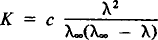# Ostwald Dilution Law

## Ostwald dilution law

[′ȯst‚vält di′lü·shən ‚lȯ]
(physical chemistry)
The law that for a sufficiently dilute solution of univalent electrolyte, the dissociation constant approximates a 2 c /(1 - a), where c is the concentration of electrolyte and a is the degree of dissociation.

## Ostwald Dilution Law

the relationship that expresses the dependence of electrical conductivity on concentration in a dilute solution of a binary weak electrolyte:where K is the dissociation constant of the electrolyte, c is the concentration, and λ and λ are the values of the electrical conductivities at c and at infinite dilution, respectively. The Ostwald dilution law proceeds from the law of mass action and the equation λ/λ = α, where α is the degree of dissociation.

The Ostwald dilution law was derived by W. Ostwald in 1888. Ostwald’s experimental confirmation of the law was very important to the the founding of the classical theory of electrolytic dissociation.

Site: Follow: Share:
Open / Close